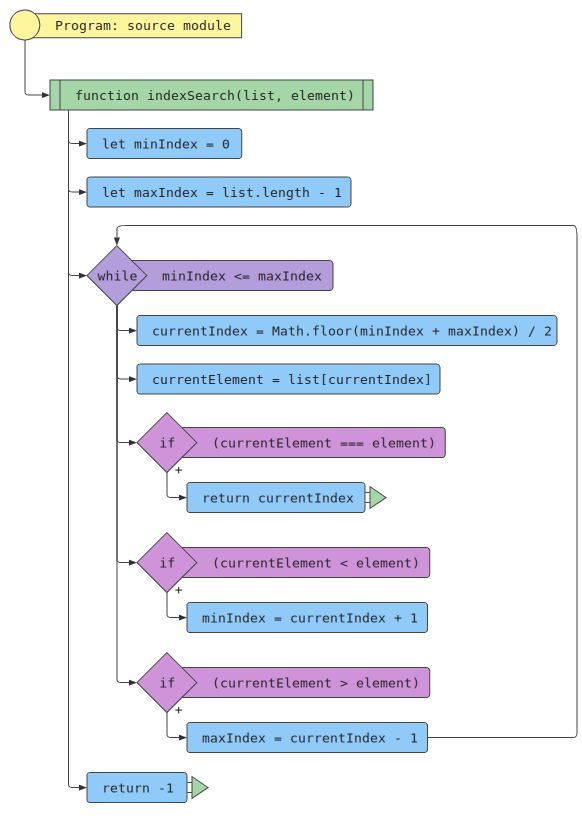JS Flowchart Pack
Share
Explore

#JS Flowchart

Generate flowcharts from JavaScript code.

JS Flowchart is a Coda Pack that allows you to pass in any Javascript code as input and receive a complete flowchart algorithm from it. The pack has a Formula called DrawFlowchart, this formula takes in JavaScript source code as a string input and outputs the algorithm of that code as an SVG image.
Javascript code input:
Here is a Javascript code function of the classic binary search algorithm.
function indexSearch(list, element) {
let currentIndex,
currentElement,
minIndex = 0,
maxIndex = list.length - 1;

while (minIndex <= maxIndex) {
currentIndex = Math.floor(minIndex + maxIndex) / 2;
currentElement = list[currentIndex];

if (currentElement === element) {
return currentIndex;
}

if (currentElement < element) {
minIndex = currentIndex + 1;
}

if (currentElement > element) {
maxIndex = currentIndex - 1;
}
}

return -1;
}
Result:
The output is a flowchart of the binary search algorithm.